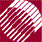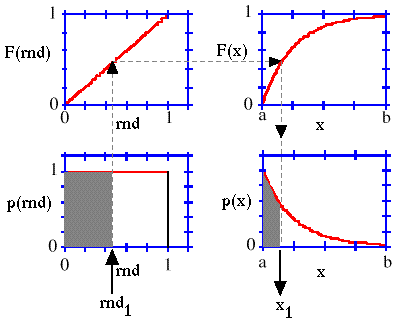ECE532 Biomedical Optics © 1998 Steven L. Jacques, Scott A. Prahl Oregon Graduate Institute

# Monte Carlo simulations: Sampling from probability density function

The Monte Carlo method is essentially a technique for sampling a probability density function based on a computer generated random number.

Consider a particular random number, rnd1. The following figure illustrates how rnd1 selects a particular value x1 from the probability density function p(x). If a large number of such x1 values were selected using a series of random numbers rnd1, the histogram of all the x1 choices, normalized by the integral of the histogram (sum of all histogram bin values times the bin widths), will match the original p(x).The next page illustrates how to use the above scheme with a specific example.

to next page | Chapter 4 | Course | Home